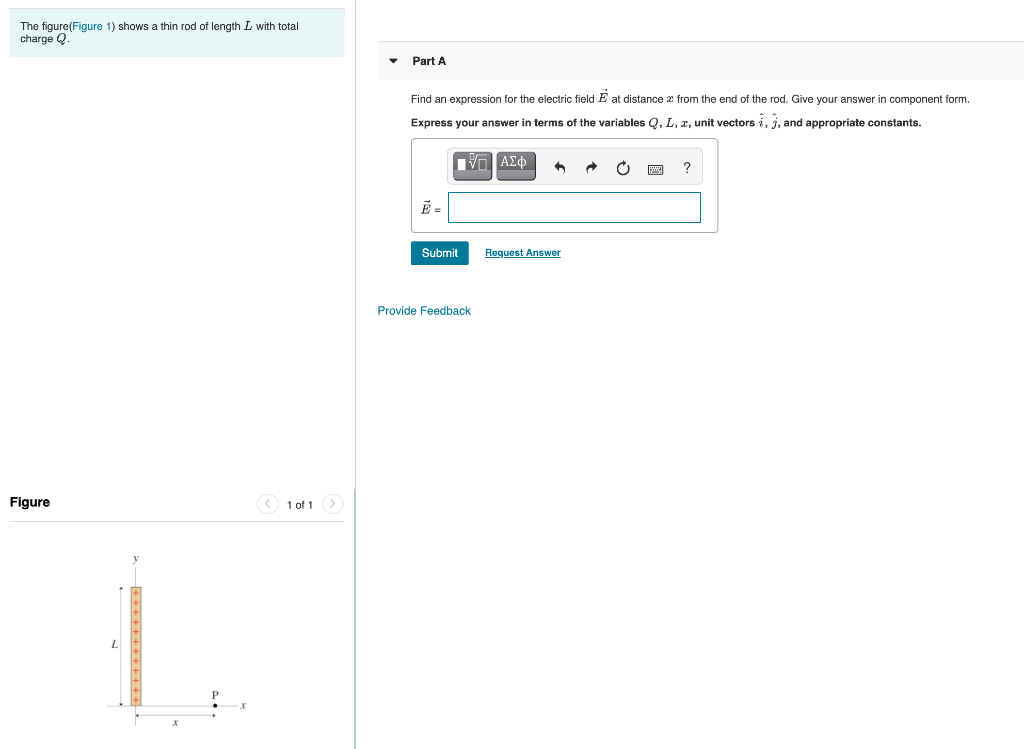# Question Solved1 Answerundefined The figure(Figure 1) shows a thin rod of length L with total charge Q Part A Find an expression for the electric field Ē at distance z from the end of the rod. Give your answer in component form. Express your answer in terms of the variables Q, L, I, unit vectors i, j, and appropriate constants. vo AEO ? Submit Request Answer Provide Feedback Figure < 1 of 1 + + + + + + + + + + + +undefined

Transcribed Image Text: The figure(Figure 1) shows a thin rod of length L with total charge Q Part A Find an expression for the electric field Ē at distance z from the end of the rod. Give your answer in component form. Express your answer in terms of the variables Q, L, I, unit vectors i, j, and appropriate constants. vo AEO ? Submit Request Answer Provide Feedback Figure < 1 of 1 + + + + + + + + + + + +
More
Transcribed Image Text: The figure(Figure 1) shows a thin rod of length L with total charge Q Part A Find an expression for the electric field Ē at distance z from the end of the rod. Give your answer in component form. Express your answer in terms of the variables Q, L, I, unit vectors i, j, and appropriate constants. vo AEO ? Submit Request Answer Provide Feedback Figure < 1 of 1 + + + + + + + + + + + +